# 谐振泵浦2640 nm Ho:YAG/SrWO4中红外拉曼激光器理论研究Theoretical Study of Resonantly Pumped Mid-Infrared Ho:YAG/SrWO4 Intracavity Raman Laserat 2640 nm

DOI: 10.12677/APP.2020.1012067, PDF, HTML, XML, 下载: 108  浏览: 175  国家自然科学基金支持

Abstract: The theoretical model of quasi-two-level Ho:YAG/SrWO4 actively Q-switched Raman laser is established according to the energy transfer process of Hoion in a resonantly pumped Ho:YAG laser. The relationship between the inverted particle density in the laser crystal, the density of the funda-mental photon and the Raman photon with time is obtained by numerical simulation. The influences of Q-switch frequency and output mirror transmittance on the average output power, pulse width, pulse energy, and peak power of the Raman laser are also analyzed, which provides a theoretical basis for achieving stable and high efficient Raman laser output at 2640 nm in future experiment investigation.

1. 引言

2 μm波段处于三个传输损耗较低的大气窗口之一，具有人眼安全特性，且可以作为3-12μm光学参量振荡器的理想泵浦源 ，因此其具有广阔的应用前景。作为该激光器的基频光增益介质，Ho:YAG晶体因其较大的发射截面和较长的上能级寿命，在2 μm中红外波段激光器的研究中备受关注  。同时，Ho3+掺杂材料具有量子缺陷较低、上转换损耗较低等优点，也为Ho:YAG固体激光器的稳定高效运转奠定了基础 。随着高质量晶体的发展，在现有的拉曼晶体中，SrWO4因其高拉曼增益系数、高光学损伤阈值、良好的机械性能和热性能等优点在近红外波段内得到了广泛的研究   。2 μm波段激光在激光医疗、化学传感、激光测距和环境监测等领域都有非常重要的应用  ，但目前2 μm波段基于SrWO4晶体的拉曼激光器鲜有报道。

2. Ho:YAG/SrWO4拉曼激光器速率方程模型的建立

2.1. Ho:YAG激光器的运转机制

1908 nm激光泵浦的Ho:YAG激光器在常温运转过程中可以视作是准二能级激光系统。在基质晶体场的作用下，Ho:YAG中掺杂的Ho3+会发生Stark能级分裂，形成多重态Stark能级 。Ho:YAG激光器的运转机制如图1所示，基态能级5I8上的Ho3+吸收1908 nm的泵浦光跃迁到激发态的Stark能级，当实现粒子数反转后，激发态能级5I7上的Ho3+向基态Stark能级跃迁并发出2122 nm激光。由于泵浦终态能级就是激光的上能级，而激光的终态能级正位于基态能级上，因此整个系统在运转过程中量子亏损极小，激光器可以高效运行。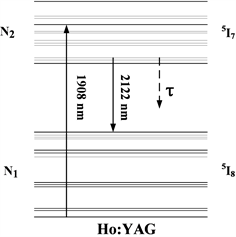Figure 1. Diagram of energy level transition process about Ho:YAG crystalinoperation process about Ho:YAG Laser

2.2. Ho:YAG/SrWO4拉曼激光器速率方程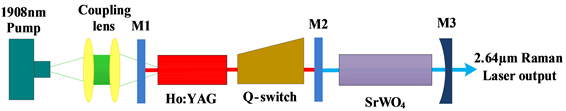Figure 2. Diagram ofexperimentalsetup

${N}_{\text{Ho}}={N}_{1}+{N}_{2}$ (1)

$\Delta N={f}_{u}{N}_{2}-{f}_{l}{N}_{1}$ (2)

$\frac{\text{d}{N}_{1}}{\text{d}t}=\frac{\text{d}{N}_{\text{Ho}}}{\text{d}t}-\frac{\text{d}{N}_{\text{2}}}{\text{d}t}=-\frac{\text{d}{N}_{2}}{\text{d}t}$ (3)

$\frac{\text{d}{N}_{2}}{\text{d}t}=R-\frac{c\sigma }{n}\Delta N{\Phi }_{L}-\frac{{N}_{2}-{N}_{2}^{0}}{\tau }$ (4)

$\frac{\text{d}{\Phi }_{L}}{\text{d}t}=\frac{c}{2{L}_{r}}\cdot \text{2}\sigma \Delta N{\Phi }_{L}{n}_{L}{l}_{L}-\frac{{\Phi }_{L}}{{t}_{L}}$ (5)

$\frac{\text{d}\Delta N}{\text{d}t}=-\left(1+{f}_{l}/{f}_{u}\right)c\sigma \Delta N{\Phi }_{L}-\frac{\Delta N}{\tau }$ (6)

$\frac{\text{d}\Delta N}{\text{d}t}=-\gamma c\sigma \Delta N{\Phi }_{L}-\frac{\Delta N}{\tau }$ (7)

$\frac{\text{d}{\Phi }_{L}}{\text{d}t}=\frac{c}{2{L}_{r}}\cdot 2\sigma \Delta N{\Phi }_{L}{n}_{L}{l}_{L}-\frac{c}{2{L}_{S}}G{\Phi }_{L}{\Phi }_{S}-\frac{{\Phi }_{L}}{{t}_{L}}$ (8)

$\frac{\text{d}{\Phi }_{S}}{\text{d}t}=\frac{c}{2{L}_{S}}G{\Phi }_{L}{\Phi }_{S}-\frac{{\Phi }_{S}}{{t}_{S}}$ (9)

$\Delta {N}^{0}=\left({f}_{u}+{f}_{l}\right)R\tau \left[1-\mathrm{exp}\left(-\frac{1}{{f}_{p}\tau }\right)\right]-{f}_{l}{N}_{\text{Ho}}$ (10)

$R=\frac{{P}_{in}{\eta }_{\alpha }{\eta }_{p}}{\pi {\omega }_{p}^{2}{l}_{L}h{\nu }_{p}}$ (11)

${P}_{peak}=\frac{1}{2{t}_{S}}h{\nu }_{S}\pi {\omega }_{S}^{2}{l}_{S}{\eta }_{c}\cdot {\Phi }_{S\text{_}\mathrm{max}}$ (12)

${\eta }_{c}=\frac{T}{T+\delta }$ (13)Table 1. Rate equation’s model parameters of Ho: YAG/SrWO4Raman Laser

3. 仿真结果与分析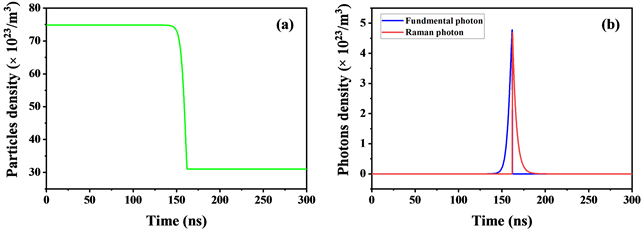Figure 3. (a) Populationinversion density and (b) fundamental photon density and Raman photondensity

3.1. Q开关重复频率对激光器输出特性的影响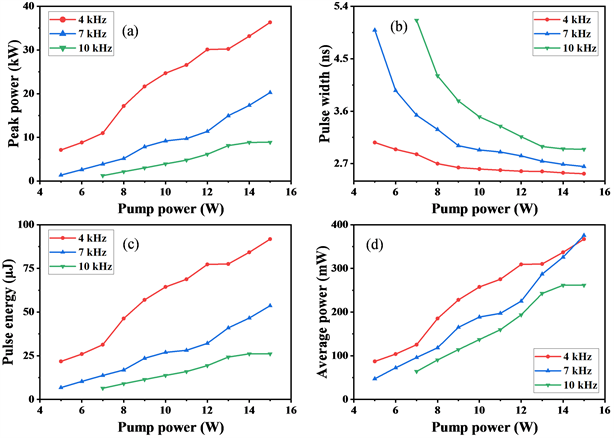Figure 4. The changing of (a) peak power, (b) pulse width, (c) pulse energy and (d) average output power with pump power under different Q-switch frequenciesFigure 5. The changing of (a) peak power, (b) pulse width, (c) pulse energy and (d) average output power with Q-switch frequency under different pump powers

3.2. 输出镜透过率的变化对激光器输出特性的影响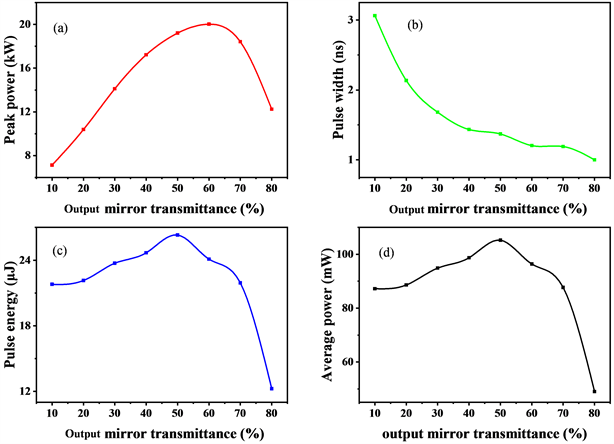Figure 6. The changing of output characteristics under different transmittance from of output mirror: (a) pulse width, (b) average output power, (c) peak power, and (d) pulse energy

4. 结论

NOTES

*通讯作者。

  Yao, B.Q., Shen, Y.J., Duan, X.M., Dai, T.Y., Ju, Y.L. and Wang, Y.Z. (2014) A 41-W ZnGeP2 Optical Parametric Os-cillator Pumped by a Q-Switched Ho:YAG Laser. Optics Letters, 39, 6589-6592. https://doi.org/10.1364/OL.39.006589  Yuan, J.H., Duan, X.M., Yao, B.Q., Li, J., Cui, Z., Shen, Y.J., Dai, T.Y., Ju, Y.L., Li, Y.C., Kou, H.M. and Pan, Y.B. (2015) Dual-End-Pumped High-Power Cr2+:ZnS Passively Q-Switched Ho:YAG Ceramic Laser. Applied Physics B, 119, 381-385. https://doi.org/10.1007/s00340-015-6086-0  Chen, H., Shen, D.Y., Zhang, J., Yang, H., Tang, D.Y., Zhao, T. and Yang, X.F. (2011) In-Band Pumped Highly Efficient Ho:YAG Ceramic Laser with 21 W Output Power at 2097 nm. Optics Letters, 36, 1575-1577. https://doi.org/10.1364/OL.36.001575  Duan, X.M., Shen, Y.J., Yao, B.Q. and Wang, Y.Z. (2018) A 106W Q-Switched Ho:YAG Laser with Single Crystal. Optik, 169, 224-227. https://doi.org/10.1016/j.ijleo.2018.05.094  Brenier, A., Jia, G.H. and Tu, C.Y. (2004) Raman Lasers at 1.171 and 1.517 μm with Self-Frequency Conversion in SrWO4:Nd3+ Crystal. Journal of Physics Condensed Matter, 16, 9103-9108. https://doi.org/10.1088/0953-8984/16/49/025  Fan, Y.X., Liu, Y., Duan, Y.H., Wang, Q., Fan, L., Wang, H.T., Jia, G.H. and Tu, C.Y. (2008) High-Efficiency Eye-Safe Intracavity Raman Laser at 1531 nm with SrWO4 Crystal. Ap-plied Physics B, 93, 327-330. https://doi.org/10.1007/s00340-008-3165-5  Errandonea, D., Tu, C., Jia, G., Martín, I.R., Rodríguez-Mendoza, U.R., Lahoz, F., Torres, M.E. and Lavín, V. (2008) Effect of Pressure on the Luminescence Properties of Nd3+ Doped SrWO4 Laser Crystal. Journal of Alloys & Compounds, 451, 212-214. https://doi.org/10.1016/j.jallcom.2007.04.180  王古常, 孙斌, 万强, 程勇. 军用脉冲激光测距技术与研究现状[J]. 光学与光电技术, 2003(4): 55-59.  Mcdaniel, S.A., Berry, P.A., Cook, G., Zelmon, D., Meissner, S., Meissner, H. and Mu, X.D. (2017) CW and Passively Q-Switched Operation of a Ho:YAG Waveguide Laser. Optics & Laser Technology, 91, 1-6. https://doi.org/10.1016/j.optlastec.2016.12.004  Zhang, X.L., Ni, K.B., Huang, J., Dong, G.Z. and Li, G.X. (2020) Resonantly Pumped Mid-Infrared Ho:YAG/BaWO4 Intracavity Raman Laserat 2640 nm. Optics and Laser Technology, 121, Article ID: 105813. https://doi.org/10.1016/j.optlastec.2019.105813  丁双红. 全固态拉曼激光器理论与实验研究[D]: [博士学位论文]. 济南: 山东大学, 2006.  陈晓寒. 1.18微米全固态拉曼激光器的高效运转及人眼安全拉曼激光器[D]: [博士学位论文]. 济南: 山东大学, 2009.  朱国利. 高重频Ho:YAG激光器及其泵浦源Tm:YLF激光器的研究[D]: [博士学位论文]. 哈尔滨: 哈尔滨工业大学, 2012.  段小明. 常温氧化物基质单掺Ho固体激光器的研究[D]: [博士学位论文]. 哈尔滨: 哈尔滨工业大学, 2012.  Zhang, X.L., Ding, Y., Qiao, Y., Li, G.X. and Cui, J.H. (2015) Diode-End-Pumped Efficient 2533 nm Intracavity Raman Laser with High Peak Power. Optics Communica-tions, 355, 433-437. https://doi.org/10.1016/j.optcom.2015.07.008  Kuzucu, O. (2015) Watt-Level, Mid-Infrared Output from a BaWO4 External-Cavity Raman Laser at 2.6 μm. Optics Letters, 40, 5078-5081. https://doi.org/10.1364/OL.40.005078  骆勇. Ho:YAG/YVO4拉曼激光器输出特性研究[D]: [硕士论文]. 哈尔滨: 哈尔滨工程大学, 2018.  Degnan, J.J. (1989) Theory of the Optimally Coupled Q-Switched Laser. IEEE Journal of Quantum Electronics, 25, 214-220. https://doi.org/10.1109/3.16265  W∙克希耐尔. 固体激光工程[M]. 孙文, 等, 译. 北京: 科学出版社, 2002: 89.